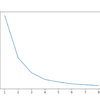# Archive | Python Machine Learning## How to Develop LARS Regression Models in Python

Regression is a modeling task that involves predicting a numeric value given an input. Linear regression is the standard algorithm for regression that assumes a linear relationship between inputs and the target variable. An extension to linear regression involves adding penalties to the loss function during training that encourage simpler models that have smaller coefficient […]## Nearest Shrunken Centroids With Python

Nearest Centroids is a linear classification machine learning algorithm. It involves predicting a class label for new examples based on which class-based centroid the example is closest to from the training dataset. The Nearest Shrunken Centroids algorithm is an extension that involves shifting class-based centroids toward the centroid of the entire training dataset and removing […]## How to Develop LASSO Regression Models in Python

Regression is a modeling task that involves predicting a numeric value given an input. Linear regression is the standard algorithm for regression that assumes a linear relationship between inputs and the target variable. An extension to linear regression invokes adding penalties to the loss function during training that encourages simpler models that have smaller coefficient […]## How to Develop Ridge Regression Models in Python

Regression is a modeling task that involves predicting a numeric value given an input. Linear regression is the standard algorithm for regression that assumes a linear relationship between inputs and the target variable. An extension to linear regression invokes adding penalties to the loss function during training that encourages simpler models that have smaller coefficient […]## How to Develop Elastic Net Regression Models in Python

Regression is a modeling task that involves predicting a numeric value given an input. Linear regression is the standard algorithm for regression that assumes a linear relationship between inputs and the target variable. An extension to linear regression involves adding penalties to the loss function during training that encourage simpler models that have smaller coefficient […]## Robust Regression for Machine Learning in Python

Regression is a modeling task that involves predicting a numerical value given an input. Algorithms used for regression tasks are also referred to as “regression” algorithms, with the most widely known and perhaps most successful being linear regression. Linear regression fits a line or hyperplane that best describes the linear relationship between inputs and the […]## Gaussian Processes for Classification With Python

The Gaussian Processes Classifier is a classification machine learning algorithm. Gaussian Processes are a generalization of the Gaussian probability distribution and can be used as the basis for sophisticated non-parametric machine learning algorithms for classification and regression. They are a type of kernel model, like SVMs, and unlike SVMs, they are capable of predicting highly […]## Radius Neighbors Classifier Algorithm With Python

Radius Neighbors Classifier is a classification machine learning algorithm. It is an extension to the k-nearest neighbors algorithm that makes predictions using all examples in the radius of a new example rather than the k-closest neighbors. As such, the radius-based approach to selecting neighbors is more appropriate for sparse data, preventing examples that are far […]## Linear Discriminant Analysis With Python

Linear Discriminant Analysis is a linear classification machine learning algorithm. The algorithm involves developing a probabilistic model per class based on the specific distribution of observations for each input variable. A new example is then classified by calculating the conditional probability of it belonging to each class and selecting the class with the highest probability. […]## Multi-Core Machine Learning in Python With Scikit-Learn

Many computationally expensive tasks for machine learning can be made parallel by splitting the work across multiple CPU cores, referred to as multi-core processing. Common machine learning tasks that can be made parallel include training models like ensembles of decision trees, evaluating models using resampling procedures like k-fold cross-validation, and tuning model hyperparameters, such as […]